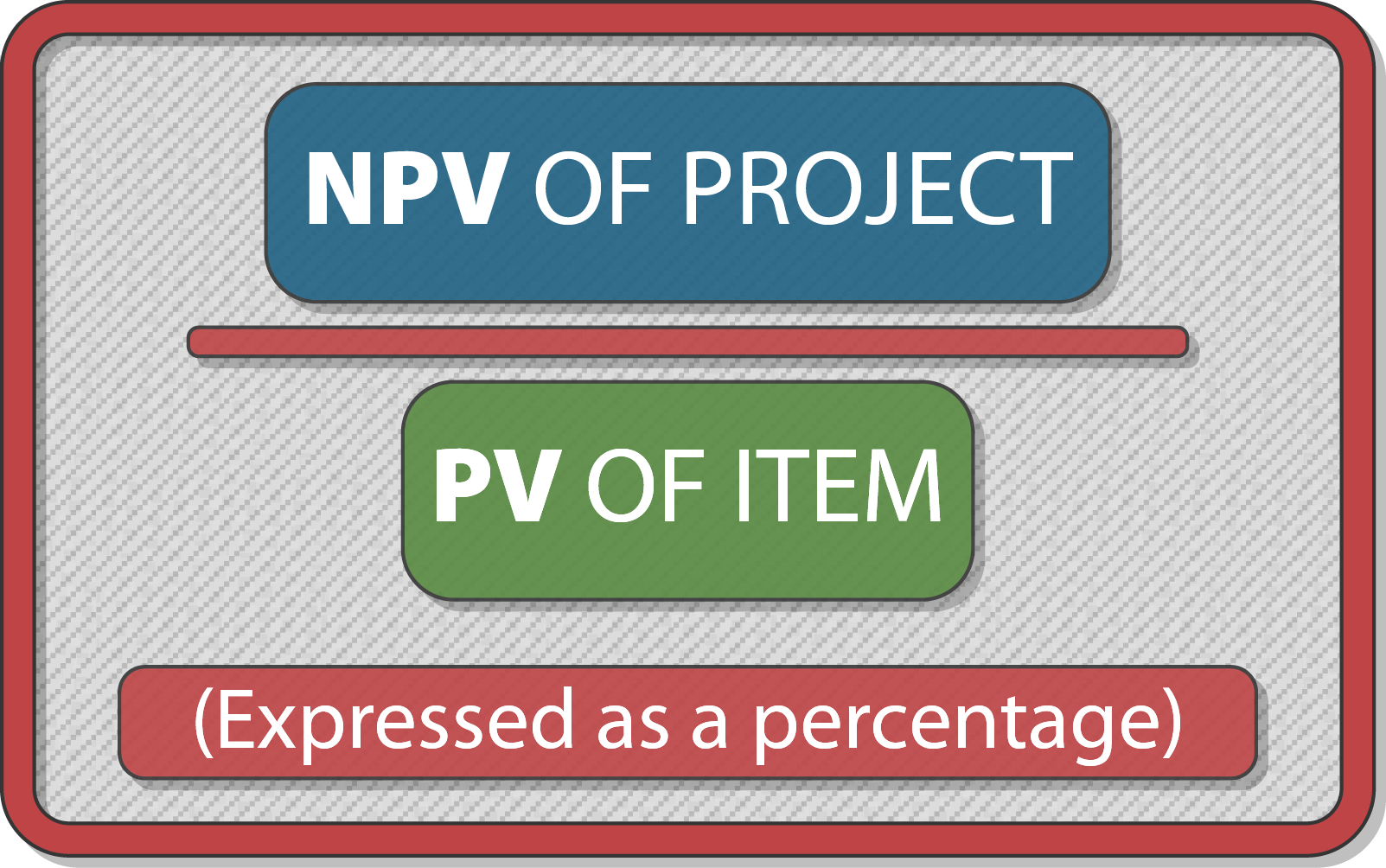FMF9
Syllabus D. Investment Appraisal D3. Adjusting for risk and uncertainty in investment appraisal

# ACCA FM F9 Syllabus D. Investment Appraisal - Sensitivity Analysis - Notes2 / 5

### How sensitive is the project to ONE particular variable

It basically shows as a % how much ONE variable has to change before the NPV becomes zero

The smaller the % change needed the more focus managers should give the item generally

#### The Calculation

•• The smaller the percentage, the more sensitive the decision is to a change in the variable

#### Illustration

ACCA colleges are considering a project which will cost them an initial 10,000

The cashflows expected for the 2 year duration are 10,000pa.

The variable costs are 1,000pa

Cost of capital 10%

Calculate the sensitivity analysis of all variables

• Solution

PV of project as a whole:

 Year 0 1 2 Investment (10,000) Costs (1,000) (1,000) Sales 10,000 10,000 Discount Factor 1 0.909 0.826 Discounted Cashflows (10,000) 8,181 7,434

So the NPV as a whole is 5,615

Sensitivity of Initial Investment
5,615 / 10,000 = 56%

Sensitivity of Costs
5,615 / (909 + 826) = 323%

Sensitivity of Sales
5,615 / (9,090 + 8,260) = 32%

#### Weakness of Sensitivity Analysis

• Each variable must change in isolation

Yet they are often interdependent upon each other

• It does not take into account probabilities of change occurring

• Some factors management may not control